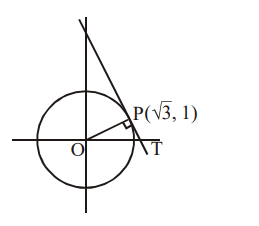# The tangent and the normal lines`
Question:

The tangent and the normal lines at the point $(\sqrt{3}, 1)$ to the circle $x^{2}+y^{2}=4$ and the $x$-axis form a triangle. The area of this triangle (in square units) is :

1. $\frac{1}{3}$

2. $\frac{4}{\sqrt{3}}$

3. $\frac{1}{\sqrt{3}}$

4. $\frac{2}{\sqrt{3}}$

Correct Option: , 4

Solution:Given $x^{2}+y^{2}=4$

equation of tangent

$\Rightarrow \sqrt{3} x+y=4$ ...........(1)

Equation of normal

$x-\sqrt{3} y=0$ ...........(2)

Coordinate of $\mathrm{T}\left(\frac{4}{\sqrt{3}}, 0\right)$

$\therefore$ Area of triangle $==\frac{2}{\sqrt{3}}$